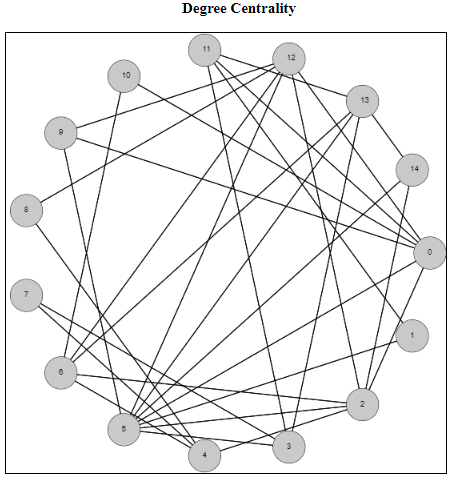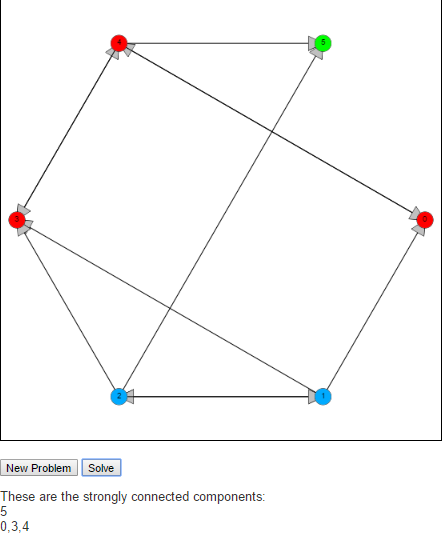# Category Archives: Scripts

These are the scripts for the algorithms featured in the examples section

# Degree Centrality of a GraphI wanted to spend some time on centrality measures of a graph. These are measurements of how important each node (or edge) is to the overall graph. But how do we define, or determine, importance? There is no unique way to answer this question, so there are varying metrics for measuring centrality. Which one you choose depends on several factors including how many other nodes of the graph are included, as well as the run time of the metrics you’re considering.

I have just published a script focusing on the degree centrality metric. The degree centrality metric is called a “walk metric” because it determines how important a node is by how many other nodes that can be reached by walks of up to a certain length. Lets look at the definition of the degree of a node to see if we can understand why it is called a walk metric.

In an undirected graph G = (V, E), the degree of a node u [in] V is the |{v | (u, v) [in] E}|. This is the size of the set of nodes that are connected to node u via a single edge. Another way of describing a single edge is a walk of length one. So the degree metric measures the importance of a node by the number of unique walks of length one.

The normalized degree centrality of a node v in a graph G = (V,E) measures how many nodes are connected to the node v, compared to the maximum possible number of edges that can be connected to this node. Because we are dealing with simple undirected graphs (at most a single edge between any two distinct vertices), this maximum possible number will always be |V – 1|. So the normalized degree can be calculated by dividing the degree of the node (the number of nodes it is connected to) by |V – 1|.

So for the example above, the node 0 has degree 6 because it is connected to nodes 2, 5, 9, 10, 11, and 12. There are 15 total nodes in this graph, so to calculate the normalized degree centrality of the node 0, it will be 6 / 14, which rounds to 0.428571.

To see more examples and to help answer questions, check out the script in my examples section on degree centrality

# Tarjan’s Strongly Connected Components AlgorithmA strongly connected component of a graph is a subgraph S of G where every pair of nodes, u and v in S there is a path from u to v and a path from v to u.

To find these strongly connected components we implement Tarjan’s algorithm. The idea behind Tarjan’s algorithm is to begin by running a depth first search from an arbitrary node in the graph, labeling nodes reachable from this start node in the order they are reached. The algorithm is also interested in the “oldest” node that could be reached by a given node. This is indicated by the keeping track of the lowest label that can be reached from that node. We will call the first property label(v) and the second lowlink(v).

When the algorithm starts label(v) is the same as lowlink(v) whenever a node is discovered. As the algorithm is executed, the DFS is being run on each discovered node, which in turn updates the lowlink(v) property telling of (older) nodes that can be reached. If an older node can be reached, then we update lowlink. If we reach a node that cannot connect to any older nodes after the DFS call, i.e if label(v) is the same a lowlink(v), then this means that this node does not have a path to any node with a lower label. So this node will be the first node of a new strongly connected component.

Feel free to check it out an let me know what you think in the comments below.